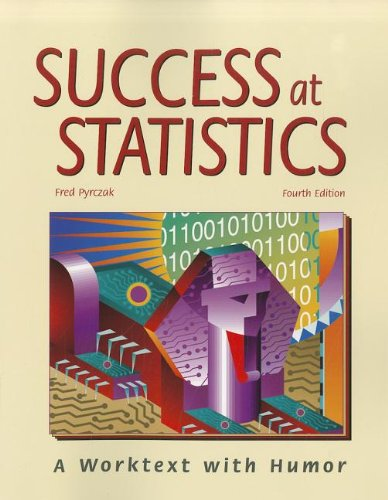• Free Shipping on all orders in the US
• Over 7 million books in stock
• Proud to be B-Corp
• We aim to be carbon neutral by 2022
• Over 120,000 Trustpilot reviews
Item 1 of 0# Success at Statistics by Fred Pyrczak

Condition - Very Good
\$6.39
Out of stock

# Faster Shipping

Get this product faster with free shipping from our US warehouse

## Success at Statistics Summary

### Success at Statistics: A Worktext with Humor by Fred Pyrczak

* This comprehensive text covers all the traditional topics in a first-semester course.

* Divided into 67 short sections, this book makes the topics easy to digest. Students regularly get positive reinforcement as they check their mastery with exercises at the end of each section.

* Each exercise is based on a humorous riddle. If the answer to a riddle makes sense, students know all their answers for that exercise are correct. If not, they know they need to check their answers.

* Short sections make it easy to customize your course by assigning only those sections needed to fulfill your objectives.

* A comprehensive basic math review at the end of this book may be used to help students whose math skills are rusty.

* Thoroughly field-tested for student interest and comprehension. The short sections and humor-based, self-checking riddles are greatly appreciated by students.

* Contains Part D on effect size, which provides technical solutions to issues raised in Part C (such as the limitations of inferential statistics).

Part A: Descriptive Statistics

1. Why is the Study of Statistics Important?

2. Descriptive Versus Inferential Statistics

3. Scales of Measurement

4. Frequencies, Percentages, and Proportions

5. Introduction to Frequency Distributions

6. Frequency Distribution for Grouped Data

7. Cumulative Frequencies, Cumulative Percentages, and Percentile Ranks

8. Histograms

9. Frequency Polygons

10. Shapes of Distributions

11. Introduction to Summation

12. The Mean: An Average

13. Mean, Median, and Mode

14. Variability: The Range and Interquartile Range

15. Variability: Introduction to the Standard Deviation

16. A Closer Look at the Standard Deviation

17. Another Look at the Standard Deviation

18. Standard Scores

19. Transformed Standard Scores

20. Standard Scores and the Normal Curve

21. Conceptual Introduction to Correlation

22. Scattergrams

Part B: Inferential Statistics

23. Introduction to Sampling

24. A Closer Look at Sampling

25. Introduction to Probability

26. Probability and the Normal Curve

27. Percentiles and the Normal Curve

28. Standard Error of the Mean

29. Confidence Interval for the Mean

30. Appropriate Figures

31. Introduction to the Null Hypothesis

32. Decisions About the Null Hypothesis

33. Introduction to the Pearson r

34. Computation of the Pearson r

35. Significance of a Pearson r

36. Coefficient of Determination

37. Multiple Correlation

38. Introduction to Linear Regression

39. Computations for Linear Regression

40. z Test for One Sample

41. When to Reject the Null Hypothesis

42. One-Tailed Versus Two-Tailed Tests

43. Introduction to the HSD Test

44. Computation of t for Independent Data

45. Reporting the Results of t Tests

46. Computation of t for Dependent Data

47. Introduction to Analysis of Variance

48. Computations for a One-Way ANOVA

49. Tukey's HSD Test

50. Scheffe's Test

51. Introduction to Two-Way ANOVA

52. Significance of the Difference Between Variances

53. Introduction to Chi-Square

54. Computations for a One-Way Chi-Square

55. Computations for a Two-Way Chi-Square

56. Cramer's Phi

57. Median Test

58. Mann-Whitney U Test

59. Wilcoxon's Matched-Pairs Test

Part C: Putting It All Together

60. Descriptive Statistics: Their Value in Research

61. Inferential Statistics: Their Value in Research

62. Limitations of Inferential Statistics: I

63. Limitations of Inferential Statistics: II

64. Statistical Versus Practical Significance

Part D: Effect

65. Introduction to Effect Size (d)

66. Interpretation of Effect Size (d)

67. Effect Size and Correlation (r)

Part E: Supplement

Basic Math Review for Statistics Students

Appendices

A. Computational Formulas for the Standard Deviation

B. Notes on Interpreting the Pearson r

C. Definition Formula for the Pearson r

D. Spearman's rho

E. Standard Error of Estimate

F. Standard Error of a Median and a Percentage

G. Confidence Interval for the Mean: Small Samples

H. Computation of the Precise Median

I. A Closer Look At Effect Size

J. Summary of Statistical Equations

Tables

1. Table of the Normal Curve

2. Table of Random Numbers

3. Constants Based on t for Computing Confidence Intervals for the Mean Based on Small Samples

4. Critical Values of t for Two-Tailed t Test

5. Critical Values of t for One-Tailed t Test

6. Critical Values of F for the .05 Level

7. Critical Values of F for the .01 Level

8. Studentized Range Statistic (q) for the .05 Level

9. Studentized Range Statistic (q) for the .01 Level

10. Minimum Values for Significance of a Pearson r

11. Critical Values of Chi-Square

12. Critical Values of U for the .05 Level

13. Critical Values of U for the .01 Level

14. Critical Values of T for Wilcoxon's Matched-Pairs Test

Index

CIN000237811
9781884585814
1884585817
Success at Statistics: A Worktext with Humor by Fred Pyrczak
Used - Very Good
Paperback
Pyrczak Publishing
2009-01-01
462
N/A
Book picture is for illustrative purposes only, actual binding, cover or edition may vary.
This is a used book - there is no escaping the fact it has been read by someone else and it will show signs of wear and previous use. Overall we expect it to be in very good condition, but if you are not entirely satisfied please get in touch with us